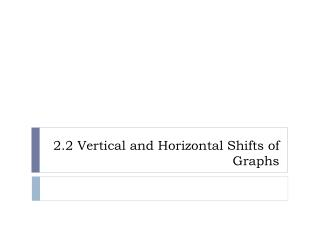DownloadDownload Presentation2.2 Vertical and Horizontal Shifts of Graphs

2.2 Vertical and Horizontal Shifts of Graphs

Download Presentation2.2 Vertical and Horizontal Shifts of Graphs

- - - - - - - - - - - - - - - - - - - - - - - - - - - E N D - - - - - - - - - - - - - - - - - - - - - - - - - - -
Presentation Transcript

1. 2.2 Vertical and Horizontal Shifts of Graphs

2. Quiz • Identify the basic function with a graph as below:

3. Vertical Shift of graphs • Discussion 1 y f(x) = x2 f(x) = x2+1 ↑ 1 unit f(x) = x2-2 ↓ 2 unit x f(x) = x2-5 ↓ 5 unit What about shift f(x) up by 10 unit? shift f(x) down by 10 unit?

4. Vertical Shift of Graphs • Discussion 2 y f(x) = x3 f(x) = x3+2 ↑ 2 unit f(x) = x3-3 ↓ 3 unit x

5. Vertical Shift of Graphs • If c>0, then the graph of y = f(x) + c is obtained by shifting the graph of y = f(x) upward a distance of c units. The graph of y = f(x) – c is obtained by shifting the graph of y = f(x) downward a distance of c units. ↑f(x) + c ↓f(x) - c

6. Horizontal Shift of graphs • Discussion 1 y f(x) = x2 f(x) = (x+1)2 ← 1 unit f(x) = (x-2)2 → 2 unit x f(x) = (x-5)2 → 5 unit What about shift f(x) left by 10 unit? shift f(x) right by 10 unit?

7. Horizontal Shift of Graphs • Discussion 2 y f(x) = |x| f(x) = |x + 2| ← 2 unit f(x) = |x - 3| → 3 unit x

8. Horizontal Shift of Graphs • If c > 0, the graph of y = f(x + c) is obtained by shifting the graph of y = f(x) to the left a distance of c units. The graph of y = f(x - c) is obtained by shifting the graph of y = f(x) to the right a distance of c units. f(x + c) ← →f(x - c)

9. Conclusion y f(x) + c ↑f(x) + c x f(x) f(x + c) ← →f(x - c) f(x + c) f(x - c) ↓f(x) - c f(x) - c

10. Combinations of vertical and horizontal shifts • Equation  write a description y1 = |x - 4|+ 3. Describe the transformation of f(x) = |x|. Identify the domain / range for both. answer: shifting f(x) up by 3 units, then shift f(x) right by 4 units. ( or shift f(x) right by 4 units, then shift f(x) up by 3 units.)

11. Combinations of vertical and horizontal shifts • Description  equation Write the function that shifts y = x2 two units left and one unit up. answer: y1 = (x+2)2+1

12. Combinations of vertical and horizontal shifts • Graph  equation Write the equation for the graph below. Assume each grid mark is a single unit. Answer: f(x) = (x-1)3-2 y x

13. Combinations of vertical and horizontal shifts • Equation  graph Sketch the graph of y = f(x) = √x-2 -1. How does the transformation affect the domain and range? y x Step 1: f(x) = √x Step 2: f(x) = √x-2 Step 3: f(x) = √x-2 -1

14. Combinations of vertical and horizontal shifts • Graph & symbolic transformation  new graph Using the given graph of f(x), sketch the graph of f(x) +2 f(x+2) f(x-1) - 3 y x

15. Math 101 schedule changes • 1)  Project 1 will be a take-home project instead of an in-class group project.  The project will be posted by Wednesday, February 9, through the MyKAPInfo link.  It is due in class on Monday, February 14.2)  Exam 1 for Math 101 will be moved from Feb 15/16 to Feb 16/17.  Group A is scheduled for Wednesday, February 16 and Group B on Thursday, February 17.  The hours for testing for both days are 7:30 am - 9:00 pm.  All exams are in ST 324.3)  Correspondingly, the deadline for full credit for Skills Test #1 is moved to Tuesday, February 15.

16. Homework • PG. 99: 3-45(M3), 47-65(odds) • KEY: 18, 27, 49, 51 • Reading: 2.3 Stretch, Shrink & Reflect